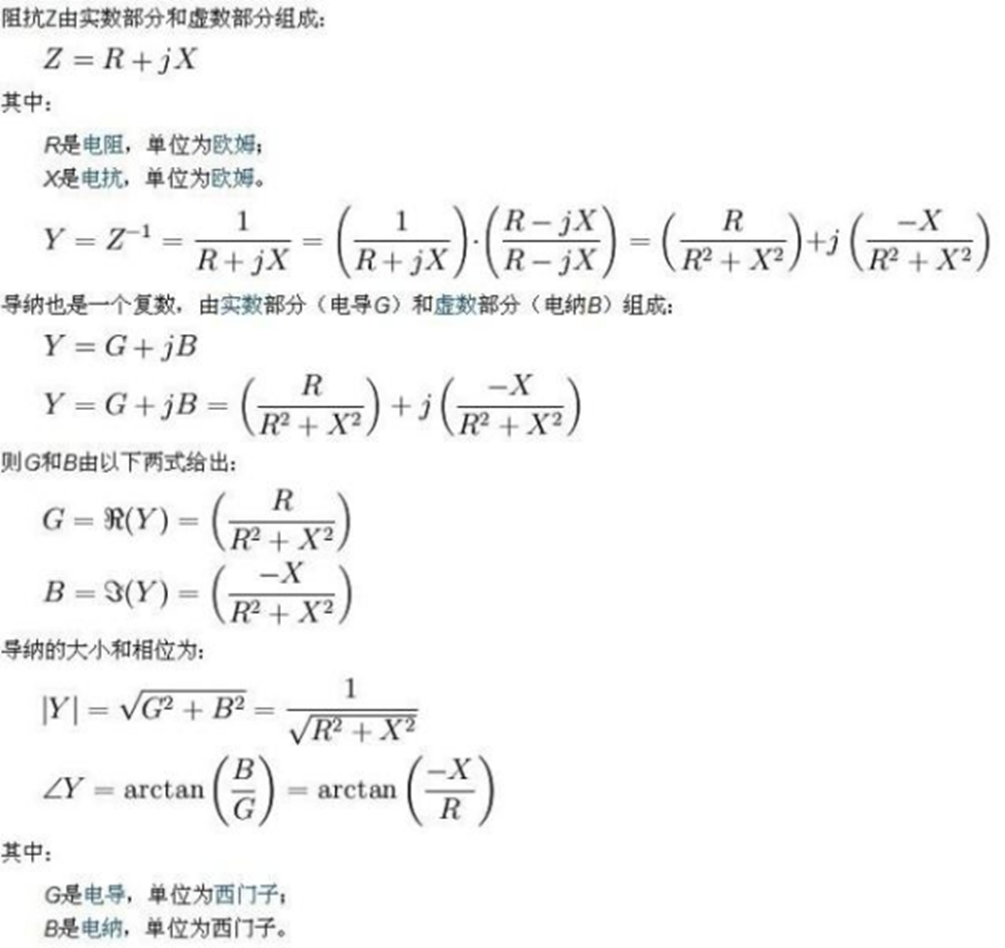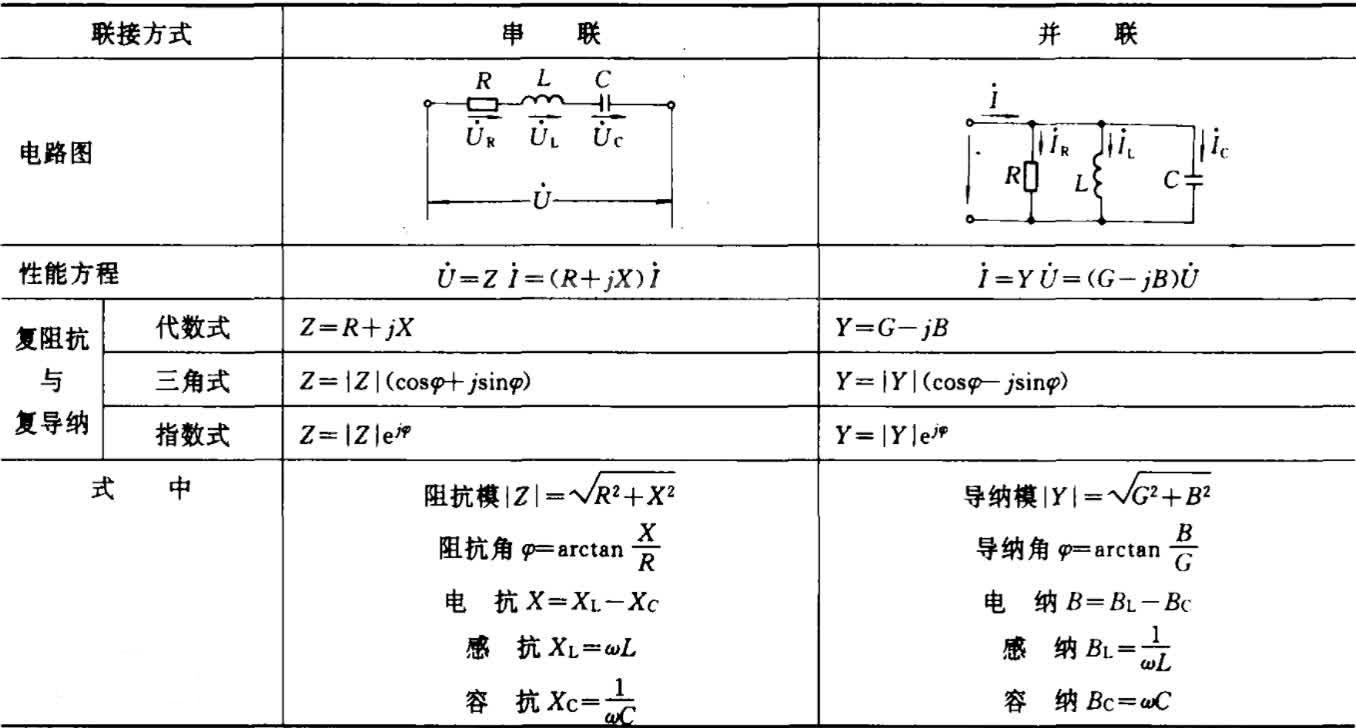Admittance is the collective term for conductance and susceptance. In power electronics, admittance is defined as the reciprocal of impedance, the symbol Y, the unit is Siemens, and West (S) for short. Like impedance, admittance is also a complex number, consisting of a real part (conductance G) and an imaginary part (susceptance B): Y = G + jB.

contents

• 1. What does admittance mean
• 2. The relationship between admittance and impedance

## 1. What does admittance mean

Admittance is the amount of energy released by the system when the voltage changes. Admittance is used to describe the difficulty of alternating current passing through a Circuit or system. Use an imaginary number to express susceptance, and the unit is Siemens. Its value is equal to the ratio of the effective value of the current and the effective value of the Voltage at the input. That is, Y=I/U, so admittance is the reciprocal of impedance.## 2. The relationship between admittance and impedance

Impedance and admittance are reciprocal relationships, and the conversion relationship is impedance = 1 part of admittance, and admittance = 1 part of impedance.